# Scales in music

In this small article I will show you some ways of thinking about the scales in music. There is a lot of rubbish floating around on the Web that contains incorrect calculations etc. My aim is to explain the concepts in both an understandable and precise way.

definition 1: A scale is a finite sequence of points that divides an octave in parts.

definition 2: The chromatic note system is the standard note system in the western world. An octave is divided into 12 notes, each a half step apart.

For illustration, let's see some scales visualized on a guitar or a bass fretboard. Think of the E string. The green boxes indicate notes that belong to the scale while the white boxes indicate the notes that do not belong to the scale. If you think about the real instruments, please note that the fretboards shown here are not drawn using correct proportions.

major (or ionian) scale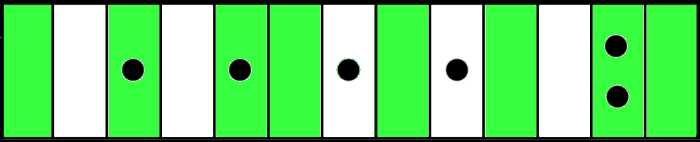mixolydian scale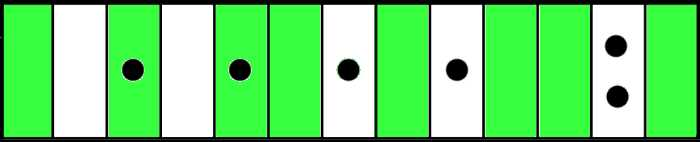natural minor (or aeolian) scale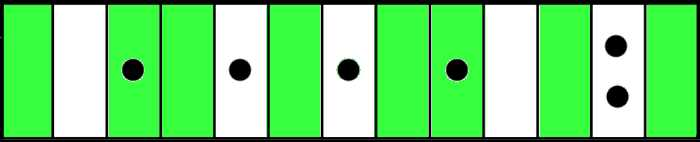harmonic minor scale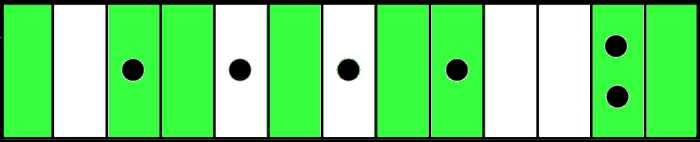blues scale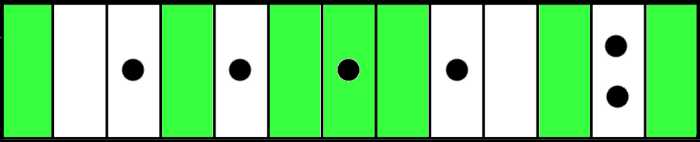Now, using the chromatic note system, how many ways are there to divide an octave? If you examine the scale shapes above, you can see that each of them contains the same starting note (i.e. the tonic) and the ending note (i.e. the nearest octave following the tonic). This is a direct consequence of our definition 1. Because we are dividing an octave, we must always include the tonic and the next octave.

octave interval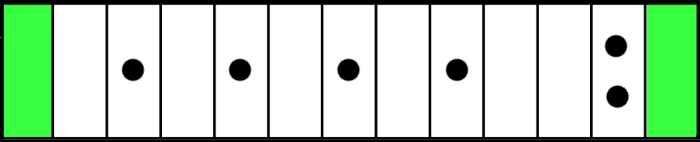In these examples, assuming standard E tuning, the tonic is on the 1st fret and so it is F, but that fact is not important to our investigation. We could start using any note and it would make no difference. This is because all the tonalities are isomorphic, i.e. the structures of octaves are always the same.

Because the starting notes and the ending notes are always included, to find the different combinations, it suffices to examine only the inner blocks of the fretboard. We proceed to take the following two steps:

• instead of using colours green and white, we use binary digits 1 and 0 to signify whether a note belongs to a scale or not
• drop the starting note and the ending note

For example, using the binary notation, the above scales and the octave interval look like this. Note that the starting and the ending note have not been dropped yet:

 major 1010110101011 mixolydian 1010110101101 natural minor 1011010110101 harmonic minor 1011010110011 blues 1001011100101 octave interval 1000000000001

Below we can see the scales after dropping the first and last binary digit. Note how the length of all the binary digit strings goes down from 13 to 11:

 major 01011010101 mixolydian 01011010110 natural minor 01101011010 harmonic minor 01101011001 blues 00101110010

Now, the important realization is this: the binary digit strings of length 11 correspond exactly to the white area of the octave interval above.

We can easily count the number of all binary strings having length 11, using the familiar formula: 2 to the power of 11. The result is 2048.

However, there is one problem. Our count includes the string 00000000000, but when we add the starting note and the ending to it, we get 1000000000001. That is the octave interval, and it is probably best to exclude it, because an interval is, well, just an interval, and perhaps not a proper scale. So to get the correct result, we count 2048 - 1 to arrive at the final result: There are 2047 different ways to divide an octave using the chromatic note system, and so 2047 different scales. Of course only a small part of these scales has been named.

What is sound? According to a physical definition, it is vibration in the air at certain wavelength, the frequency of which is measured using Hertz or Hz. For example, the tuning, or pitch, of a certain A note could be 440Hz, 441Hz or 442Hz, meaning that many vibrations per second. We will now ignore the fact that natural sounds consist of not only the base frequency, but of additional harmonic multiples too.

Assuming a 440Hz tonic A, the octave from that A to another higher pitched A is the frequency range between 440Hz and 880Hz. It is important to understand that the chromatic note system is only one possible way of splitting the octave. What if we included quarter steps, like they do in some non-western cultures? How many scales would we have then?

definition 3: The quarter note system is an alternative way of dividing an octave into parts. In this system the notes are a quarter step apart.

Quarter note fretboard with 26 frets shown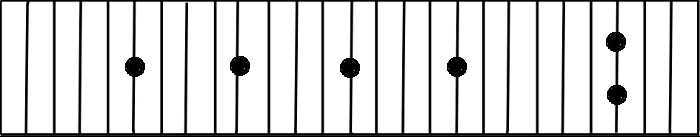Now the octave from F to F would look like this:

Quarter note fretboard octave from F to F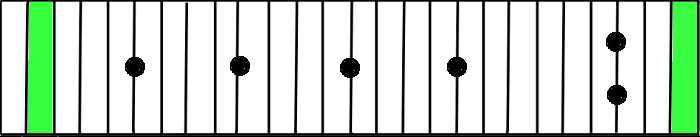Using the same argument as with the chromatic note system, we will now investigate only the white blocks inside the green blocks. We have 23 white blocks, hence all our binary digit strings will be 23 digits long. So we count 2 to the power of 23, getting 8388608. And just like we ignored the all-zeroes bit string of length 11, we can also ignore the 00000000000000000000000 of length 23. So our final result concerning the quarter note system is that there are 8388608 - 1, 8388607 different ways and thus 8388607 scales in there. That is a huge amount, almost 8 and a half million combinations, especially when compared to our earlier chromatic system result.